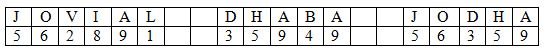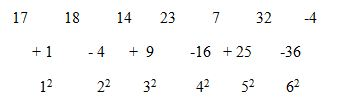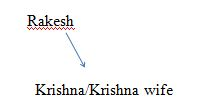# SSC CGL 2018 Practice Test Papers | Reasoning Ability (Day-10)

Dear Aspirants, Here we have given the Important SSC CGL Exam 2018 Practice Test Papers. Candidates those who are preparing for SSC CGL 2018 can practice these questions to get more confidence to Crack SSC CGL 2018 Examination.

[WpProQuiz 2777]

Click “Start Quiz” to attend these Questions and view Explanation

1. If JOVIAL is coded as562891, and DHABA is coded as 35949, then JODHA is coded as
1. 3514372
2. 3154372
3. 3154732
4. 3157432

2. Find the number marked as? in the series given below.

17, 18, 14, ? , 7, 32, -4

1. 10
2. 15
3. 14
4. 12

3. Find odd one out

1. Mist
2. Fog
3. Smog
4. Sultry

4. Which of the following conclusion does not follow?

Statement

L>T, T<W, W=M; A≥H; M>H

1. L < M
2. T > M
3. W> H
4. None of the above

5. A, B and C are statements such that if both A and B are true, then C is false. Further A is always true. Then—

1. B is always false
2. If C is true, then B is false
3. C is always true
4. If C is false, then B is true

6. Select the related word/letters/number from the given alternatives.

35:195:: ?? : 255

a.93

b.36

c.63

d.72

7.A series is given with one term missing. Choose the correct alternative from the given ones that will complete the series.

80, 105, 165, ??

a.250

b.230

c.240

d.235

8. If ‘−’ stands for ‘×’, ‘×’ stands for ‘+’, ‘+’ stands for ‘÷’, and ‘÷’ stands for ‘−’, then 15 + 6 – 7 ÷ 3 × 12 =?

1. 26
2. 40.5
3. 33
4. 26.5

9. The father of Krishna is the only son of Rakesh’s father. How is wife of krishna related to Rakesh?

1. Mother
2. Daughter in law
3. Sister
4. Daughter
1. If the letters of the given word are used as many times as one wants to use which of the words given below can be formed?

IRRIGATION

1. Nation
2. Red
3. Lion
4. RatioExcept d other 3 prevails in cold climate.

35 = 62-1

195 = 142-1

63 = 82-1

255 = 162-1

Difference series:

80+25 = 105; 105+50 = 165; 165+75 = 240

The only son of Rakesh’s father is rakesh himself.